• 对于频域图像增强
万次阅读
2020-10-29 01:27:11

人类传递信息的主要媒介是语言和图像。据统计在人类接受的各种信息中视觉信息占80%，所以图像信息是十分重要的信息传递媒体和方式。图像传递系统包括图像采集、图像压缩、图像编码、图像存储、图像通信、图像显示这六个部分。在实际应用中每个部分都有可能导致图像品质变差，使图像传递的信息无法被正常读取和识别。例如，在采集图像过程中由于光照环境或物体表面反光等原因造成图像整体光照不均，或是图像采集系统在采集过程中由于机械设备的缘故无法避免的加入采集噪声，或是图像显示设备的局限性造成图像显示层次感降低或颜色减少等等。因此研究快速且有效地图像增强算法成为推动图像分析和图像理解领域发展的关键内容之一。

图像增强处理是数字图像处理的一个重要分支。很多由于场景条件的影响图像拍摄的视觉效果不佳，这就需要图像增强技术来改善人的视觉效果，比如突出图像中目标物体的某些特点、从数字图像中提取目标物的特征参数等等，这些都有利于对图像中目标的识别、跟踪和理解。图像增强处理主要内容是突出图像中感兴趣的部分，减弱或去除不需要的信息。这样使有用信息得到加强，从而得到一种更加实用的图像或者转换成一种更适合人或机器进行分析处理的图像。图像增强的应用领域也十分广阔并涉及各种类型的图像。例如，在军事应用中，增强红外图像提取我方感兴趣的敌军目标；在医学应用中，增强X射线所拍摄的患者脑部、胸部图像确定病症的准确位置；在空间应用中，对用太空照相机传来的月球图片进行增强处理改善图像的质量；在农业应用中，增强遥感图像了解农作物的分布；在交通应用中，对大雾天气图像进行增强，加强车牌、路标等重要信息进行识别；在数码相机中，增强彩色图像可以减少光线不均、颜色失真等造成的图像退化现象。

图像工程是一门综合学科，它的研究内容非常广泛，覆盖面也很大。从1996年起，《中国图像图形学报》上连续刊登了对图像工程文献统计分类的综述文章。根据各文献的主要内容将其分别归入图像处理、图像分析、图像理解、技术应用和综述5个大类，并在此基础上对国内15种有关图像工程的重要中文期刊进行了各期刊各类文献的统计和分析。选取的刊物名有:《CT理论与应用研究》、《测绘学报》、《电子测量与仪器学报》、《电子学报》、《电子与信息学报》、《计算机学报》、《模式识别与人工智能》、《数据采集与处理》、《通信学报》、《信号处理》、《遥感学报》、《中国生物医学工程学报》、《中国体视学与图像分析》、《中国图象图形学报》、《自动化学报》。

从中我们挑选了最近5年的统计数据：在2005年的112期上发表的2734 篇学术研究和技术应用文献中，属于图像工程领域的文献有656篇。在2006年

的112期上发表的3013篇学术研究和技术应用文献中，属于图像工程领域的文献有711篇。在2007年的118期上发表的3312篇学术强究和技术应用文献中，属于图像工程领域的文献有895篇。在2008年的120期上发表的3359篇学术研究和技术应用文献中，属于图像工程领域的文献有915篇，2009年的134期上发表的3604篇学术研究和技术应用文献中，有1008篇属于图像工程领域的文献。这些统计数据显示，无论是论文总数还是选取总数都是逐年增长的。论文总数的增长表明刊物的不断发展，选取总数的增加表明图像工程的研究和应用的不断壮大。据统计从1995年至2009年，发表图像处理的文章总计2720篇，占图像工程总体的33.1%；发表图像分析的文章总计2434篇，占图像工程总体的29.6%；发表图像理解的文章总计1192篇，占图像工程总体的14.5%；发表技术应用文章1797篇，占图像工程总体的21.9%；发表综述评论文章74篇，占图像工程总体的0.9%，其中关于图像增强技术方面的文章增长率尤其较高。因此图像增强技术在今后一段时间内仍将是一个热点。

影响图像质量清晰程度有很多因素，室外光照度不均匀会造成图像灰度过于集中；摄像头获得的图像经过数/模转换，线路传输时都会产生噪声污染，图像质量不可避免降低，轻者变现为图像伴有噪点，难于看清图像细节；重者图像模糊不清，连大概物体面貌轮廓都难以看清。因此，对图像进行分析处理之前，必须对图像进行改善，即增强图像。图像增强并不考虑图像质量下降的原因，只是将图像中感兴趣的重要特征有选择性的突出出来，同时衰减不需要的特征，目的就是提高图像的可懂度。

图像增强的方法分为空域法和频域法两种，空域法是对图像中的像素点进行操作，用公式描述如下：

G(x,y)=F(x,y) H(x,y)

其中是F(x,y)原图像；H(x,y)为空间转换函数；G(x,y)表示进行处理后的图像。

频域法是间接的处理方法，是先在图像的频域中对图像的变换值进行操作，然后变回空域。例如,先对图像进行傅里叶变化到频域，再对图像的频谱进行某种滤波修正，最后将修正后的图像进行傅里叶反变化到空域，以此增强图像。可用图1来描述该过程

# 第二章 频域图像的原理

## 2.1 引言

在进行图像处理的过程中，获取原始图像后，首先需要对图像进行预处理，因为在获取图像的过程中，往往会发生图像失真，使所得图像与原图像有某种程度上的差别。在许多情况下，人们难以确切了解引起图像降质的具体物理过程及

其数学模型，但却能估计出使图像降质的一些可能原因，针对这些原因采取简单易行的方法，改善图像质量。图像增强一般不能增加原图像信息，只能针对一些成像条件，把弱信号突出出来，使一些信息更容易分辨。图像增强的方法分为频域法和空域法，空域法主要是对图像中的各像素点进行操作；而频域法是在图像的某个变换域内，修改变换后的系数，例如傅立叶变换、DCT 变换等的系数，对

图像进行操作，然后再进行反变换得到处理后的图像。

MATLAB矩阵实验室（Matrix Laboratory）的简称，具有方便的数据可视化功能，可用于科学计算和工程绘图。它不仅在一般数据可视化软件都具有的功能方面更加完善，而且对于一些其他软件所没有的功能（例如图形的光照处理、色度处理以及四维数据的表现等），MATLAB同样表现了出色的处理能力。它具有功能丰富的工具箱，不但能够进行信号处理、语音处理、数值运算，而且能够完成各种图像处理功能。本文利用MATLAB工具来研究图像频域增强技术。图像增强是为了获得更好质量的图像，通过各种方法对图像进行处理，例如图像边缘检测、分割以及特征提取等技术。图像增强的方法有频域处理法与空域处理法，本文主要研究了频域处理方法中的滤波技术。从低通滤波、高通滤波、同态滤波三个方面比较了图像增强的效果。文章首先分析了它们的原理，然后通过MATLAB软件分别用这三种方法对图像进行处理，处理后使图像的对比度得到了明显的改善，增强了图像的视觉效果。

## 2.2  频域增强定义和步骤

图像增强技术基本上可分成两大类：频域处理法和空域处理法。频域处理法的基础是卷积定理，它采用修改图像傅立叶变换的方法实现对图像的增强处理。在频域空间，图像的信息表现为不同频率分量的组合。如果能让某个范围内的分量或某些频率的分量受到抑制而让其他分量不受影响，就可以改变输出图的频率分布，达到不同的增强目的。

频域增强是利用图像变换方法将原来的图像空间中的图像以某种形式转换到其它空间中，然后利用该空间的特有性质方便地进行图像处理，最后再转换回原来的图像空间中，从而得到处理后的图像

频域增强的主要步骤是：

(1) 选择变换方法，将输入图像变换到频域空间；

(2) 在频域空间中，根据处理目的设计一个转移函数并进行处理；

(3) 将所得结果用反变换得到图像增强

卷积理论是频域技术的基础。设函数f（x,y）与线性位不变算子h（x,y）的卷积结果是g（x,y），即g(x,y)=h(x,y)*f(x,y)

那么根据卷积定理在频域有：

G(x,y)=H(u,v)F(u,v)

其中G(x,y)、 H(u,v)、F(u,v)分别是g(x,y)、h(x,y)、f(x,y)的傅立叶变换。

（4）技术所需增强图的傅立叶变换。

（5）将其与一个（根据需要设计的）转移函数相乘。

（6）再将结果进行傅立叶反变换以得到增强的图。

（7）将图像从空域转换到频域所需的变换及将图像从频域空间转换回空域

所需的变换。

（8）在频域空间对图像进行增强加工操作。

常用的频域增强方法有低通滤波和高通滤波。以下分别介绍在MATLAB中如何实现。

## 同态滤波

一般来说，图像的边缘和噪声都对应于傅立叶变换的高频分量。而低频分量主要决定图像在平滑区域中总体灰度级的显示，故被低通滤波的图像比原图像少一些尖锐的细节部分。同样，被高通滤波的图像在图像的平滑区域中将减少一些灰度级的变化并突出细节部分。为了增强图像细节的同时尽量保留图像的低频分量，使用同态滤波方法 可以保留图像原貌的同时，对图像细节增强.。滤波后，如图（2）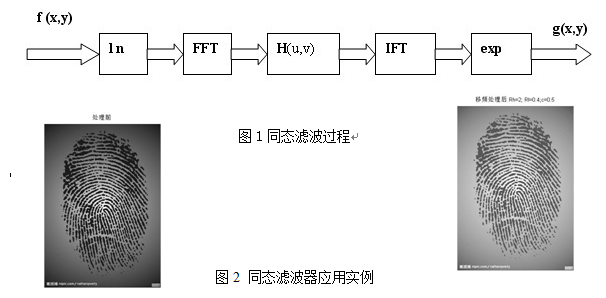图1同态滤波过程

图2 同态滤波器应用实例

同态滤波函数的确定：

以Rh 代表高频增益, Rl 代表低频增益, D( u,v ) 表示频率( u, v) 距滤波器中心( u0, v 0) 的离。传统的同态滤波函数如图2 所示。当Rh> 1, Rl> 1 时,该滤波函数能同时抑制照明分量和增强反射分量,从而满足动态范围压缩和对比度增强两种效果要求。处理目的不同, 可构造不同的滤波器。频域内经常使用的高通滤波器为高斯型高通滤波器, 滤波函数为：

H ( u, v) = 1- ex p[ - D( u, v ) / 2D0^2n]

增强处理。对高斯型高通滤波器稍加修改, 可得以下高斯型高通滤波函数:

H ( u, v) = ( Rh- R l) [ 1- e- c( D (u ,v ) / D0) 2n] + Rl

D( u, v) = [ ( u- M/ 2)^ 2+ ( v- N / 2)^2]^1/2

式中: D0 是u0 和v 0 为0 时的D( u, v ) 值, 表示截止频率。对于一幅M×N 的图像, 傅里叶变换后的中心在( M/ 2, N / 2) 式需做平移处理。传统的巴特沃思高通滤波器与同态滤波的传递函数十分相似, 巴特沃思高通滤波的传

递函数为

H ( u, v) = 1/ ( 1+ D0 / D( u, v) )^ 2n

根据两者之间的相似性, 将高通滤波函数稍作修改即可得到对应的巴特沃思高通滤波函数:

H ( u, v) = ( Rh- R l) / ( 1+ cD 0/ D( u, v ) )^2n+ Rl

# 频域增强算法的实现及代码

## 4.1  理想低通滤波器

本次设计是用低通滤波为例，图像中的边缘和噪声都对应图像傅立叶变换中的高频部分，所以如要在频域中消弱其影响就要设法减弱这部分频率的分量。根据要求我们需要选择1个合适的H(u,v)以得到消弱F(u,v)高频分量的G(u,v).在以下讨论中我们考虑对F(u,v)的实部和虚部影响完全相同的滤波转移函数。具有这种特性的滤波器称为零相移滤波器。理想是指小于D0的频率可以完全不受影响地通过滤波器，而大于D0的频率则完全通不过。1个2-D理想低通滤波器的转移函数满足下列条件：

\* MERGEFORMAT

(4-1)

上式中D0是1个非负整数。D(u,v)是从点（u,v）到频率平面原点的距离，D(u,v)=(u2+v2)1/2。

图1给出H的1个剖面图（设D对原点对称），这里理想是指小于D0的频率可以完全不受影响地通过滤波器，而大于D0的频率则完全通不过。因此D0也叫截断频率。尽管理想低通滤波器在数学上定义得很清楚，在计算机模拟中也可实现，但在截断频率处直上直下的理想低通滤波器是不能用实际的电子器件实现的。

图1 理想低通滤波器转移函数的剖面图

如果使用这些“非物理”的理想滤波器，其输出图像会变得模糊和有“振铃（ring）”现像出现。我们可借助卷积定理解释如下。

为简便，考虑1-D的情况。对1个理想低通滤波器，其h(x)的一般形式可由求式（1）的傅立叶反变换得到，其曲线可见图2（a）。现设f(x)是1副只有1个亮像素的简单图像，见图2（b）。这个亮点可看作是1个脉冲的近似。在这种情况下，f(x)和h(x)的卷积实际上是把h(x)复制到f(x)中亮点的位置。比较图2（b）和图2（c）可明显看出卷积使原来清晰的点被模糊函数模糊了。对更为复杂的原始图，如我们认为其中每个灰度值不为零的点都可以看作是1个其值正比于该点灰度值的1个亮点，则上述结论仍可成立。

图2空间模糊示意图

由图2还可以看出h(x,y)在2-D图像平面上将显示出一系列同心圆环。如对1个理想低通滤波器的H(u,v)求反变换，则可知道h(x,y)中同心圆环的半径是反比于D0的值的。所以如果D0较小，就会使h(x,y)产生数量较少但较宽的同心圆环，并使g(x,y)模糊得比较厉害。当增加D0时，就会使h(x,y)产生数量较多但较窄的同心圆环，并使g(x,y)模糊得比较少。如果D0超出F(u,v)的定义域，则h(x,y)在其对应的空间区域为1，h(x,y)与f(x,y)的卷积仍是f(x,y)，这相

当于没有滤波。

程序代码如下：

figure,imshow(I1)

I2=imnoise(I1,'salt');

figure,imshow(I2)

f=double(I2);

k=fft2(f);

g=fftshift(k);

[N1,N2]=size(g);

n=2;

d0=50;

n1=round(N1/2);

n2=round(N2/2);

for i=1:N1

for j=1:N2

d=sqrt((i-n1)^2+(j-n2)^2);

if d<=d0 h=1;else h=0;

end

y(i,j)=h*g(i,j);

end

end

y=ifftshift(y);

m=ifft2(y);

n=uint8(real(m));

figure,imshow(n)

原图

加噪后的图像

去噪后的图像

由实验结果图可以看出加噪图像是使原始图像按照指定的噪声参数加入噪声，经过低通滤波后，去噪图像的椒盐噪声被模糊处理，产生模糊和振铃现象，此振铃现象会随着截止频率的不同而变化。同时人的图像信息也被模糊化，因而效果并不是很好。

## 4.2   巴特沃斯低通滤波器

物理上可以实现的一种低通滤波器是巴特沃斯（Butterworth）低通滤波器。在进行图像处理的过程中，获取原始图像后，首先需要对图像进行图像预处理，因为在获取图像的过程中，往往会发生图像失真，使所得图像与原图像有某种程度上的差别。在许多情况下，人们难以确切了解引起图像降质的具体物理过程及其数学模型，但却能估计出使图像降质的一些可能原因，针对这些原因采取简单易行的方法，改善图像质量。由于噪声、光照等原因，使图像质量不高，为了改善视觉效果或便于人、机器对图像的分析理解，一般都需要对图像进行增强处理，但这个过程并没有统一的标准。图像增强一般不能增加原图像信息，只能针对一些成像条件，把弱信号突出出来，使一些信息更容易分辨。 图像增强的方法比较多，可以大概分为对比度增强，直方图增强、平滑和锐化［1］4大类，其中，直方图均衡是图像增强的经典方法，因为其有效性和简单易用性已成为图像增强最常用的方法，他又分为全局均衡和局部均衡2种。全局的直方图均衡［2.3］是对整幅图像进行均衡，使其灰度分布均匀，让每一个灰度等级上的像素个数基本相等，算法简单、计算量小、容易实现，但对图像细节部分增强不够；局部直方图均衡［4.5］则可以增强图像内部细节信息，得到很好的增强效果。Yin［6］通过对小波分解各个分量进行直方图均衡，然后重构得到处理后图像，S．M．Pi-zer［2］提出自适应直方图均衡算法，这也是一种局部均衡算法。在对全局直方图均衡和局部直方图均衡详细分析的基础上，本文提出了一种基于Buterworth低通滤波的图像增强方法。由于Buterworth低通滤波器在抑制噪声的同时，图像边缘模糊程度大大减小，且没有振铃效应。基于以上特点，用Buterworth低通滤波器将低频分量和高频分量分离，低频分量进行均衡后，再将两部分融合，实现图像的增强。

一个阶为n，截断频率为D0的巴特沃斯低通滤波器的转移函数为

\* MERGEFORMAT

(4-2)

阶为1的巴特沃斯低通滤波器剖面示意图见图3。由图可见低通巴特沃斯滤波器在高低频率间的过渡比较光滑，所以用巴特沃斯滤波器得到的输出图其振铃效应不明显。

一般情况下，常取是H最大值降到某个百分比的频率为截止频率。在上面式中，当D(u,v)=D0时，H(u,v)=0.5(即降到50%)。另一个常用的截止频率值是使H降到最大值的1/21/2时的频率。

图3 巴特沃斯低通滤波器转移函数的剖面示意图

用MATLAB实现Butterworth低通滤波器的代码所示：

figure,imshow(I1);

f=double(I1);

k=fft2(f);

g=fftshift(k);

[N1,N2]=size(g);

n=2;

d0=50;

n1=round(N1/2);

n2=round(N2/2);

for i=1:N1

for j=1:N2

d=sqrt((i-n1)^2+(j-n2)^2)

h=1/(1+0.414*(d/d0)^(2*n));

y(i,j)=h*g(i,j);

end

end

y=ifftshift(y);

X2=ifft2(y);

X3=uint8(real(X2));

figure,imshow(X3);

由实验结果图可以看出加噪图像，经过低通滤波后，去噪图像被模糊处理，产生模糊和振铃现象，此振铃现象会随着截止频率的不同而变化。同时人的图像信息也被模糊化，因而效果并不是很好。

MATLAB实现Butterworth高通滤波器代码所示：

figure,imshow(I1)

f=double(I1);

k=fft2(f);

g=fftshift(k);

[N1,N2]=size(g);

n=2;

d0=25;

n1=fix(N1/2);

n2=fix(N2/2);

for i=1:N1

for j=1:N2

d=sqrt((i-n1)^2+(j-n2)^2);

if d==0

h=0;

else

h=1/(1+(d0/d)^(2*n));

end

y(i,j)=h*g(i,j);

end

end

y=ifftshift(y);

X2=ifft2(y);

X3=uint8(real(X2));

figure，imshow(X3)；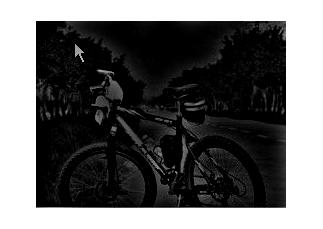原图处理后的图像

更多相关内容
• 写出不同滤波器的图像频域降噪和边缘增强算法,并进行比较，得出结论。
• 图像增强处理：设计一套空间域与频率域结合的图像增强算法，处理噪声图像，去除噪声，提高图像质量。已知：噪声为随机噪声和周期... 包括需要的图片，课设报告，MATLAB加噪代码，空域与频域结合使用的多种图像增强算法
• 频域图像增强技术，采用傅里叶变换，使用零填充的方法的滤波效果和不使用零填充的滤波的效果。
• 空域图像增强以及频域图像增强 | matlab图像处理2 提示：这里可以添加系列文章的所有文章的目录，目录需要自己手动添加 例如：第一章 Python 机器学习入门之pandas的使用 提示：写完文章后，目录可以自动生成，如何...

# 前言

图像处理基础知识也很重要，不然在matlab里键入代码就是一头雾水，今天是空域图像增强和频域图像增强</font>

# 空域图像增强

空域：指图像平面本身
【频域：对图像进行傅里叶变换等频域变换】
空域图像增强：
直接作用于像素改变其特性的增强方法

• 点操作
• 模板操作/领域操作：板操作则主要通过设计模板系数来实现不同的增强操作

图像的数据结构：uint8 ，uint16 , double

## 一.灰度映射：点处理

灰度级范围不变

### 1.图像求反（将灰度值翻转）

反转变换表达式：

						t = (L-1)-s
(L为总的灰度级数，s为当前灰度级）


matlab实例如下：

A = imread('circuit.tif');
B = 255 - A;
subplot(121);imshow(A);title('原图');
subplot(122);imshow(B);title('图像求反');


### 2.对数变换（动态范围压缩）

动态范围压缩：与增强对比度相反

		t = C log( 1 + |s| )

A = imread('circuit.tif');
c = 50
B = c*log(double(A)+1);
subplot(121);imshow(A);title('原图');
subplot(122);imshow(uint8(B));title('对数变换');


用途：扩展暗区### 3.幂次变换

			s = c*r^γ


具有对数变换相似的作用，但是更加灵活
γ>1压缩灰度
γ<1扩展灰度

f1 = imread('lena.bmp');
f = rgb2gray(f1);
minvalue = double(min(min(f)));
maxvalue = double(max(max(f)));
range = maxvalue - minvalue;
Min = 0;
Max = 255;
Range = Max - Min;
f = Range * (double(f) - minvalue)/range + Min;
%对图像进行灰度归一化实现f = mat2gray(I,[0,255]);功能
Gamma = 0.9;%设定gamma的值
s1 =double(f).^Gamma;%进行gamma变换
Gamma = 1.2;
s2 =double(f).^Gamma;
figure;
subplot(1,3,1);
imshow(uint8(f));
title('原图');
subplot(1,3,2);
imshow(uint8(s1));
title('伽玛变换（gamma=0.9）');
subplot(1,3,3);
imshow(uint8(s2));
title('伽玛变换（gamma=1.2）');


结果：γ>1压缩灰度，细节丢失
γ<1扩展灰度

### 4.阶梯量化

将图像灰度分阶段量化成较少的级数，达到数据量压缩的效果
（将图像灰度分阶段量化成较少的级数，这可在保持原图动态范围的基础上，减少灰度级数，即减少表示灰度所需的比特数，从而获得数据量压缩的效果。这里的映射是多对一的，不仅灰度值s会映射成灰度值t，而且在灰度值s前后一定范围内的灰度值也会映射成灰度值t ）

### 5.阈值切分（二值化）

大于阈值255
小于阈值 0
对比度最大但是细节全丢失了

f = imread('circuit.tif');
thresh = graythresh(f);     %自动确定二值化阈值
f1 = im2bw(f,thresh);
subplot(121);
imshow(f);
title('原图');
subplot(122);
imshow(f1);
title('二值化');（有点艺术那味了，喜欢hhh)

## 二.图像运算：集合处理

### 1.算术运算（对灰度图像）:

加减乘除
上面各运算的含义是指通过算术运算从两个像素的灰度值得到一个新的灰度值，作为对应结果新图像相同位置处像素的灰度值。新灰度值有可能超出原图像的动态范围，此时常需要进行灰度映射，以将运算结果的灰度值限制在或调整到原图像允许的动态范围内

#### 加法：

用图像平均消除随机噪声
（噪声互不相关，且具有零均值）
高斯噪声？

#### 减法：

设有图像f(x, y)和h(x, y)，对它们进行相减运算，显示两幅图像间的差异
——可用于运动检测（照明恒定，差值不为0处表示像素有移动）

## 三.直方图修正：直方图处理

1.图像的直方图：反映了图像的基本灰度级特征（暗，亮，低对比度，高对比度）
借助对图像直方图的修改或变换，可以改变图像像素的灰度分布，从而达到对图像进行增强的目的

#### 直方图均衡化

Aim: 增强动态范围偏小的图像的反差
个方法的基本思想是把原始图的直方图变换为在整个灰度范围内均匀分布的形式，这样就增加了像素灰度值的动态范围，从而可达到增强图像整体对比度的效果
累计直方图重新量化，形成基本均匀的分布

f = imread('baboon1.bmp');
f = rgb2gray(f);%灰度化
[m,n] = size(f);
GP = zeros(1,256);
for k = 0:255
GP(k+1) = length(find(f == k))/(m*n);
end
S1=zeros(1,256);
for i = 1:256
for j = 1:i
S1(i)=GP(j)+S1(i);
end
end
S2 =round((S1*256)+0.5);
for i = 1:256
GPeq(i) = sum(GP(find(S2 == i)));
end
figure;
subplot(221);
bar(0:255,GP,'b');
title('原图像直方图')
subplot(222);
bar(0:255,GPeq,'b')
title('均衡化后的直方图')
X = f;
for i = 0:255
X(find(f==i)) = S2(i+1);
end
subplot(223);
imshow(f);
title('原图像');
subplot(224);
imshow(X);
title('直方图均衡化后的图像');直方图规定化：
单映射规则SML
组映射规则GML？
咋算的？

## 四.空域滤波：模板处理

用像素及像素邻域组成的空间进行图像增强的方法

#### 线性滤波——卷积

##### 空域滤波器分类：
###### 按照效果分：

平滑滤波器：消除噪声，减弱或消除图像中高频率分量
锐化滤波器:使图像反差增强，边缘明显，减弱或消除图像中的低频率分量

###### 按运算性质分：

线性：卷积
非线性：逻辑运算，“卷积，算术，逻辑”的混合运算

#### 高斯平滑滤波器

根据高斯概率分布来确定模板的各系数值

#### 中值滤波器

非线性平滑滤波器

中值滤波器的主要功能就是让与周围像素灰度值的差比较大的像素改取与周围像素值接近的值，所以它对孤立的噪声像素的消除能力是很强的。由于它不是简单地取均值，因而产生的模糊比较少。换句话说，中值滤波器既能消除噪声又能保持图像的细节

（相比于均值滤波，中值滤波边缘轮廓比较清晰）

#### 线性锐化滤波器

线性锐化滤波器的模板仅中心系数为正而周围的系数均为负值
在图像处理中一般限制图像的灰度值为正，所以卷积锐化后还需将输出图像灰度值范围通过变换变回到原图像的灰度范围

#### 图像的梯度算子

##### 1.sobel微分算子：

一阶导数最大值寻找边界
需要x方向图像就对y求微分，需要y方向图像就对x求微

在学习的时候看到这个写的不错，贴这儿
仔细看看，最好算算

利用二阶导数为0寻找边界

• 课后习题未做

# 频域图像增强

频域增强：通过改变图像中不同频率分量实现增强，而非逐个像素处理

卷积理论是频域技术的基础。设函数f(x, y)与线性位移不变算子h(x, y)的卷积结果是g(x, y)，即g(x, y) = h(x, y) * f(x, y)
那么根据卷积定理在频域有G(u, v)=H(u, v)F(u, v)，其中G(u, v)，H(u,v)，F(u, v)分别是g(x, y)，h(x, y)，f(x, y)的傅里叶变换。用线性系统理论的话来说，H(u, v)是**转移函数
**
这样具有所需特性的g(x, y)就可得到：
g(x, y)=F–1[H(u, v)F(u, v)]
ps: u,v均为频率变量

## 1.傅里叶变换

傅里叶变换
（1）计算需增强图的傅里叶变换。
（2）将其与一个（根据需要设计的）转移函数相乘。（3）再将结果傅里叶反变换以得到增强的图。

2-D傅里叶变换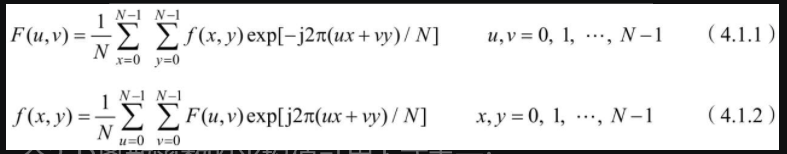一个2-D离散函数的傅里叶变换在原点的值（零频率分量）与该函数的均值成正比

卷积定理指出：两个函数在空间的卷积与它们的傅里叶变换在频域的乘积构成一对变换，而两个函数在空间的乘积与它们的傅里叶变换在频域的卷积构成一对变换

相关定理指出：两个函数在空间的相关与它们的傅里叶变换（其中一个为其复共轭）在频域的乘积构成一对变换，而两个函数（其中一个为其复共轭）在空间的乘积与它们的傅里叶变换在频域的相关构成一对变换。即[插图]如果f(x)和g(x)是同一个函数，称为自相关；如果f(x)和g(x)不是同一个函数，称为互相关
？？？？？？没懂

f = imread('circuit.tif');
f = im2gray(f);
F1 = fft2(f);%傅里叶变换
F2 = fftshift(F1);%中心化
F1m = abs(F2);
F11 = log(F2);
figure;
imshow(uint8(F1m));


## 2.频域增强基本概念

图像的傅里叶变换用F(u, v)表示，滤波器的频域函数用H(u, v)表示，增强后图像的傅里叶变换用G(u, v)表示，则要实现低通滤波就需要选择一个合适的H(u, v)以得到能减弱F(u, v)高频分量的G(u, v)

## 3.低通滤波器——频域平滑

低频通过，高频衰减，用于减少尖锐的细节变化

#### 理想低通滤波器 (ILPF)

		H(u,v) =  1, D(u,v)<Do || D(u,v)==Do
H(u,v) =  0, D(u,v)>Do


D(u, v)是从点(u, v)到频率平面原点的距离，D(u, v)=(u2+v2)1/2
大于截止频率Do（D0是一个非负整数）为0，小于等于为1

会出现振铃效应

#### 巴特沃斯低通滤波器（BLPF)

		H（u，v) = 1 / （1 + [D(u,v) / Do]^2n）


一阶无振铃，阶数越高振铃越明显

高斯低通滤波器没有振铃

## 4.高通滤波器——频域锐化

傅里叶变换中，低频主要决定图像在平滑区域中总体灰度级的显示，而高频决定图像细节部分，如边缘和噪声

高频通过，低频衰减
使图像更为锐化

滤波器函数：低通滤波器的反操作

	**H**hp(u,v) = 1 - **H**lp(u,v)


#### 理想高通滤波器 (IHPF)

		H(u,v) =  0, D(u,v)<Do || D(u,v)==Do
H(u,v) =  1, D(u,v)>Do


大于截止频率Do为1，小于等于为0

会出现振铃效应

#### 巴特沃斯高通滤波器（BHPF)

		H（u，v) =1 / （1 + [ Do / D(u,v) ]^2n）


由于高低频率之间的过渡比较光滑，所以振铃效应不明显

#### 特殊高通滤波器

##### 高通增强滤波器

通过对频域里高通滤波器的转移函数加一个常数以将一部分（本要滤除的）低频分量加回到滤波结果中，从而获得较好的视觉效果
公式

##### 高频提升滤波器

如果把原始图乘以一个放大系数A再减去低通图就可构成高频提升滤波器
公式

## 5.带通带阻与同态滤波器

#### 带阻滤波器

组织一定频率范围内的信号通过，而允许其他频率范围内的信号通过
（像把一个带子阻拦在外面）

（让这一个带子通过）

### 同态滤波器

一方面减弱图像中的低频分量（压缩了整体的动态范围），另一方面加强高频分量（增加了图像相邻各部分之间的对比度）

# 总结

## 空域技术与频域技术

空域频域
平滑低通
锐化高通
平滑滤波器的模板函数的傅里叶变换低通滤波器的转移函数
锐化滤波器的模板函数的傅里叶变换高通滤波器的转移函数
以上就是今天学习的内容，本文介绍了一些重要概念，其中关于傅里叶变换，拉普拉斯算子，属于非常重要的内容，大概会在后续再专门写写！ 然后频域图像增强也很重要，后面的带通带阻的公式后续专门写写！并且理解！
展开全文matlab 图像处理
• 1.了解图像增强的基本原理和算法；2.直方图均衡化、规定化；3.灰度图像的空域增强；4.灰度图像的频域增强；
• 利用傅里叶变换得到频域，然后将不需要的频点置零，然后进行反变换，进行滤波
• 数字图像频域增强，彩色图像频域滤波器，灰度和彩色图像的离散余弦变换。MATLAB程序
• 图像的傅里叶变换，慢速numpy实现，加速numpy实现。

### 图像的傅里叶变换

图像的频域增强其实就是：先对图像进行变换，将图像转换到变换域，然后在变换域进行操作以实现图像增强。常用的变换域就是频域（频率域，傅里叶变换的结果）。图像的频域增强有直观的物理意义。例如，图像模糊是图像中高频分量不足的结果，在频域里增加高频分量或减少低频分量就能消除一些模糊。又如，图像有时会受到重复出现的有规律周期噪声的影响，周期噪声具有特定的频率，所以可以采取频域滤波的方法滤除相应噪声频率，从而消除周期噪声。

### 傅里叶变换(2D)及其性质

f ( x , y ) → F ( u , v ) f(x, y) \to F(u, v)

### F ( u , v ) = ∑ x = 0 M − 1 ∑ y = 0 N − 1 f ( x , y ) e − j 2 π ( u x M + v y N ) F(u, v) = \sum_{x=0}^{M-1} \sum_{y=0}^{N-1} f(x,y)e^{-j2\pi(\frac{ux}{M} + \frac{vy}{N})}

• f ( x , y ) f(x, y) : 像素矩阵中 x , y x, y 处的像素值
• M , N M, N : 像素矩阵的大小，行列
• F ( x , y ) F(x, y) : 变换后 u , v u, v 处的频域值，为复数。

F ( u , v ) → f ( x , y ) F(u, v) \to f(x, y)

### f ( x , y ) = 1 M N ∑ u = 0 M − 1 ∑ v = 0 N − 1 F ( u , v ) e j 2 π ( u x M + v y N ) f(x, y) = \frac{1}{MN} \sum_{u=0}^{M-1} \sum_{v=0}^{N-1} F(u, v)e^{j2\pi(\frac{ux}{M} + \frac{vy}{N})}

F ( u , v ) = R ( u , v ) + I ( u , v ) F(u, v) = R(u, v) + I(u, v)
频谱：
∣ F ( u , v ) ∣ = ( R ( u , v ) 2 + I ( u , v ) 2 ) |F(u, v)| = \sqrt{({R(u, v)}^2 + {I(u, v)}^2)}
相位角：
ϕ = a r c t a n I ( u , v ) R ( u , v ) \phi = arctan \frac{I(u, v)}{R(u, v)}
功率谱：
P ( u , v ) = ∣ F ( u , v ) ∣ 2 = R ( u , v ) 2 + I ( u , v ) 2 P(u, v) = |F(u, v)|^2 = {R(u, v)}^2 + {I(u, v)}^2

傅里叶变换的性质：可分离性和对称性。在进行2D傅里叶变换时，可以利用其性质来简化计算，一个2D傅里叶变换核可以分解为两个1D傅里叶变换。一个 N × N N\times N 的2D傅里叶变换需要 N 4 N^4 次复数乘法运算和 N 2 ( N 2 − 1 ) N^2(N^2-1) 次复数加法运算，而进行一个长度为N的1D傅里叶变换只需进行 N 2 N^2 次复数乘法运算和 N ( N − 1 ) N(N-1) 次复数加法运算。

指数运算部分：
e − j 2 π ( u x M + v y N ) = e − j 2 π u x M + e − j 2 π v y N e^{-j2\pi(\frac{ux}{M} + \frac{vy}{N})} = e^{-j2\pi\frac{ux}{M}} + e^{-j2\pi\frac{vy}{N}}

e j 2 π ( u x M + v y N ) = e j 2 π u x M + e j 2 π v y N e^{j2\pi(\frac{ux}{M} + \frac{vy}{N})} = e^{j2\pi\frac{ux}{M}} + e^{j2\pi\frac{vy}{N}}

列变换：
G ( x , y ) = ∑ y = 0 N − 1 f ( x , y ) e − j 2 π v y N G(x, y) = \sum_{y = 0}^{N-1} f(x, y) e^{\frac{-j2\pi vy}{N}}
行变换：
F ( u , v ) = ∑ x = 0 M − 1 G ( x , y ) e − j 2 π u x M F(u, v) = \sum_{x = 0}^{M-1} G(x, y) e^{\frac{-j2\pi ux}{M}}

### 实现傅里叶变换

• 简单实现慢速傅里叶变换
• 加速版本
• numpy.fft.fft2()
import cv2 as cv
import numpy as np
import seaborn as sns
import matplotlib.pyplot as plt

img = cv.imread('./images/guigu1_mini.png')
img = cv.cvtColor(img, cv.COLOR_BGR2RGB)
img_gray = cv.cvtColor(img, cv.COLOR_RGB2GRAY)


测试用例:傅里叶变换与逆变换

def slowly_dft(img_gray):
ret = np.zeros_like(img_gray).astype('complex')
# ret应包含零频率项，
# ret[1:n//2]应该包含正频率项，
# ret[n//2 + 1:]应该包含负频率项，从最负频率开始按升序排列。
m, n = img_gray.shape
for u in range(m):
for v in range(n):
for x in range(m):
for y in range(n):
ret[u, v] += img_gray[x, y]*np.exp(-2j*np.pi*(u*x/m + v*y/n))
return ret

def slowly_idft(img_freq):
img_gray = np.zeros_like(img_freq)
m, n = img_freq.shape
for x in range(m):
for y in range(n):
for u in range(m):
for v in range(n):
img_gray[x, y] += img_freq[u, v]*np.exp(2j*np.pi*(u*x/m + v*y/n))/m*n
img_gray = np.clip(img_gray, 0, 255).astype(int)
return img_gray

def restore_img(idft_array):
real_part = idft_array.real
img_gray = np.clip(real_part, 0, 255).astype("uint8")
return img_gray

def accelerate_dft(img_gray):
ret = np.zeros_like(img_gray).astype('complex')
m, n = img_gray.shape
x_arr = np.concatenate([np.arange(m).reshape(m, 1)], axis=1)
y_arr = np.concatenate([np.arange(n).reshape(1, n)], axis=0)
for u in range(m):
for v in range(n):
ret[u, v] = np.sum(np.multiply(img_gray,np.exp(-2j*np.pi*(u*x_arr/m + v*y_arr/n))))
return ret

%%time
img_freq_slow = slowly_dft(img_gray)

CPU times: total: 1min 3s
Wall time: 1min 3s

%%time
img_freq_acce = accelerate_dft(img_gray)

CPU times: total: 1.08 s
Wall time: 1.08 s

%%time
img_freq_fast = np.fft.fft2(img_gray)

CPU times: total: 0 ns
Wall time: 0 ns


快速傅里叶逆变换

img_1 = np.fft.ifft2(img_freq_slow)
img_2 = np.fft.ifft2(img_freq_fast)


还原和频谱图（log变换,0频率移动）### 图像频域增强(OpenCV)

G ( u , v ) = H ( u , v ) F ( u , v ) G(u, v) = H(u, v)F(u, v)

• F ( u , v ) F(u, v) : 傅里叶变换后的图像频域矩阵
• H ( u , v ) H(u, v) : 转移函数，作用于 f ( u , v ) f(u, v)
• G ( u , v ) G(u, v) : 增强后的频域矩阵

图像频域增强步骤：

1. 傅里叶变换得到频域矩阵。
2. 将其与一个转移函数（根据需要设计）相乘。
3. 将结果进行傅里叶反变换以得到增强后的图像。

下通过一个简单转移操作，实现高通滤波，观察原始图像的变化：

img = cv.imread('./images/hj.png')
img = cv.cvtColor(img, cv.COLOR_BGR2RGB)
img_gray = cv.cvtColor(img, cv.COLOR_RGB2GRAY)

dft = cv.dft(img_gray.astype('float32'),flags = cv.DFT_COMPLEX_OUTPUT)           # 傅里叶变换(Opencv是用深度为2数组表示复数)
dft_shift = np.fft.fftshift(dft)                                                 # 移动零频分量
magnitude_spectrum = 20*np.log(cv.magnitude(dft_shift[:,:,0],dft_shift[:,:,1]))  # 幅值对数变换


测试用例 （逃学威龙1）从图像的幅值可以看出，中间最亮也代表着中间频率最低(幅值越大频率越小)。

##### 1. 屏蔽中间 10 × 10 10 \times 10 的低频
crow = dft_shift.shape//2                         # 中心点坐标
ccol = dft_shift.shape//2
dft_shift[crow-5:crow+5, ccol-5:ccol+5] = 0          # 去除中心低频
dft_ishift = np.fft.ifftshift(dft_shift)             # 还原频谱图
img_ = cv.idft(dft_ishift)                           # 逆傅里叶变换
img_back1 = cv.magnitude (img_[:,:,0],img_[:,:,1])   # 还原图像


屏蔽部分低频后(高通滤波)，图像得到了类似边缘检测的结果。展开全文图像增强 数字图像处理 傅里叶变换 图像频域增强
• 改善图像质量，使图像频域范畴内得到增强，采用高低通滤波器对原始图像进行一系列增强处理，并比较它们的增强效果。结果表明采用高频滤波不但会使有用的信息增强，同时也使噪声增强。相比之下，低通滤波器的处理...
• 如频谱的直流分量比例于图像的平均亮度、噪音对应于频率较高的区域、图像实体对应于频率较低的区域频域增强原理在频谱中，图像中心处对应了图像中变化较平缓的区域，四周对应了图像边缘、噪音或者变化陡峭的部分。...

### 1. 频域增强原理

在频域中，变换系数反映了某些图像的特征。如频谱的直流分量比例于图像的平均亮度、噪音对应于频率较高的区域、图像实体对应于频率较低的区域

在频谱中，图像中心处对应了图像中变化较平缓的区域，四周对应了图像边缘、噪音或者变化陡峭的部分。因此我们可以将代表了图像边缘、噪音或者变化陡峭的高频率成分滤除掉，只留下变化平缓的低频率成分，再由频域变换回时域，这就相当于对图像进行了平滑处理；相反，若滤去低频率成分，只留下高频率成分，就相当于对图像进行了锐化处理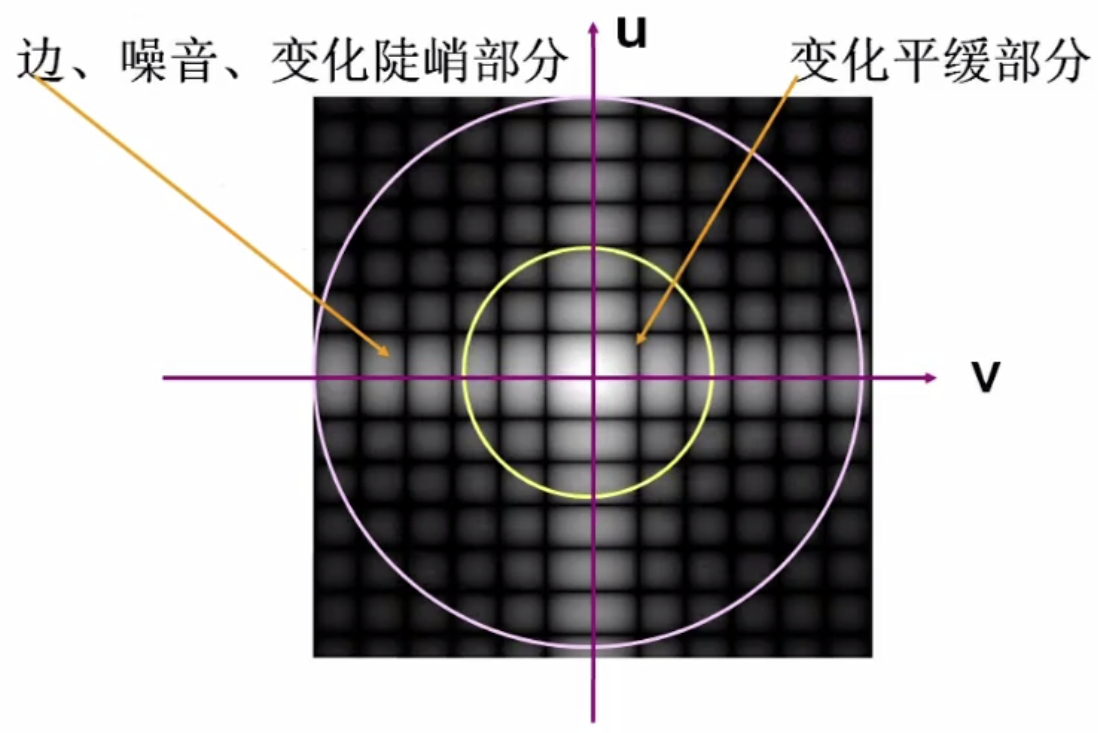可以看出，频域增强的原理十分简单，其应用的重点问题在于滤波半径阈值如何选取。

### 2. 低通滤波器

有卷积定理，滤波数学表达式为： G ( μ , ν ) = F ( μ , ν ) H ( μ , ν ) G(\mu,\nu)=F(\mu,\nu)H(\mu,\nu) 滤波后的图像为： G ( x , y ) = f − 1 { G ( μ , ν ) } = f − 1 { F ( μ , ν ) H ( μ , ν ) } G(x,y)=f^{-1}\{G(\mu,\nu)\}=f^{-1}\{F(\mu,\nu)H(\mu,\nu)\} 其中 H ( μ , ν ) H(\mu,\nu) 即为冲激响应的变换形式，低通滤波器设计的关键就在于 H ( μ , ν ) H(\mu,\nu)

几种低通滤波器

##### 2.1. 理想圆形低通滤波器 (Ideal Circular Low Pass Filter)

理想圆形低通滤波器是在傅里叶平面上半径为 D D 的圆形滤波器，其冲激响应为 H ( μ , ν ) = { 1 , D ( μ , ν ) ⩽ D 0 0 , D ( μ , ν ) ⩾ D 0 ， D 0 为 截 止 频 率 H(\mu,\nu)=\begin{cases} 1, & D(\mu,\nu)\leqslant D_0 \\[2ex] 0, & D(\mu,\nu)\geqslant D_0 \end{cases}，D_0为截止频率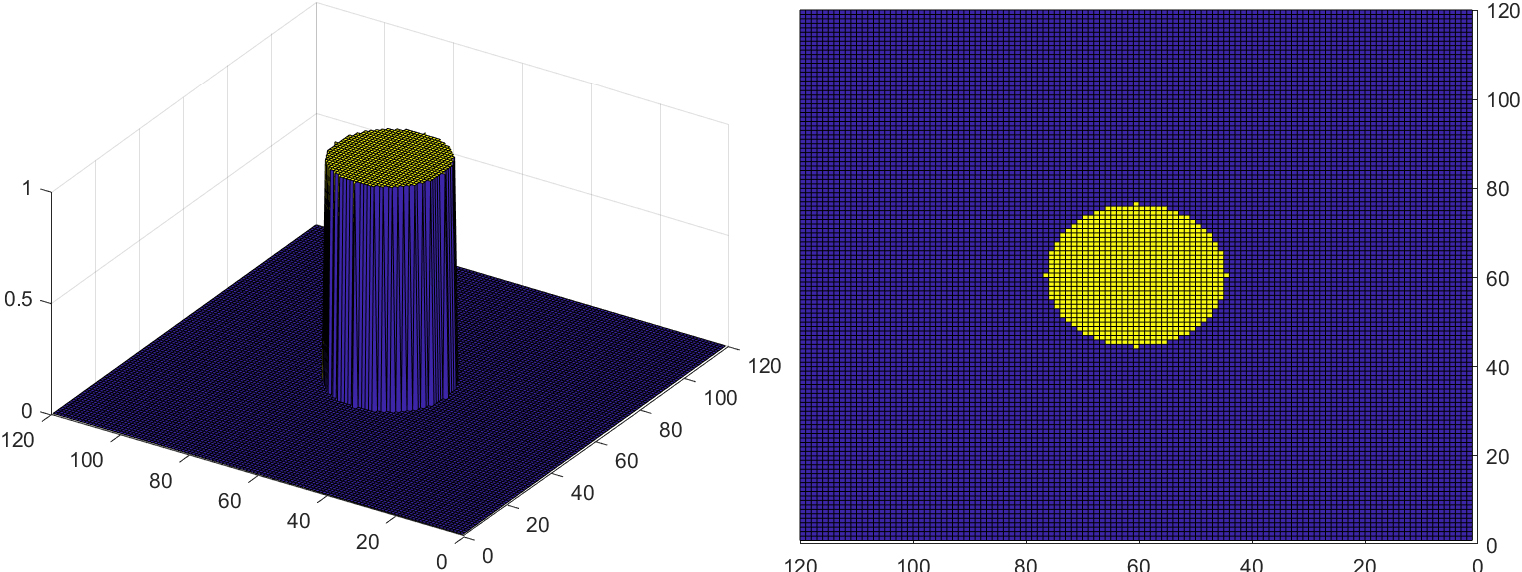理论上来说， D 0 D_0 半径内的频率分量无损通过，而圆外的频率分量会被滤除，但由于其自身会产生振铃效应，常常导致实际结果并不理想

振铃效应示意图：
可以看到在空域图像外围有剧烈的震荡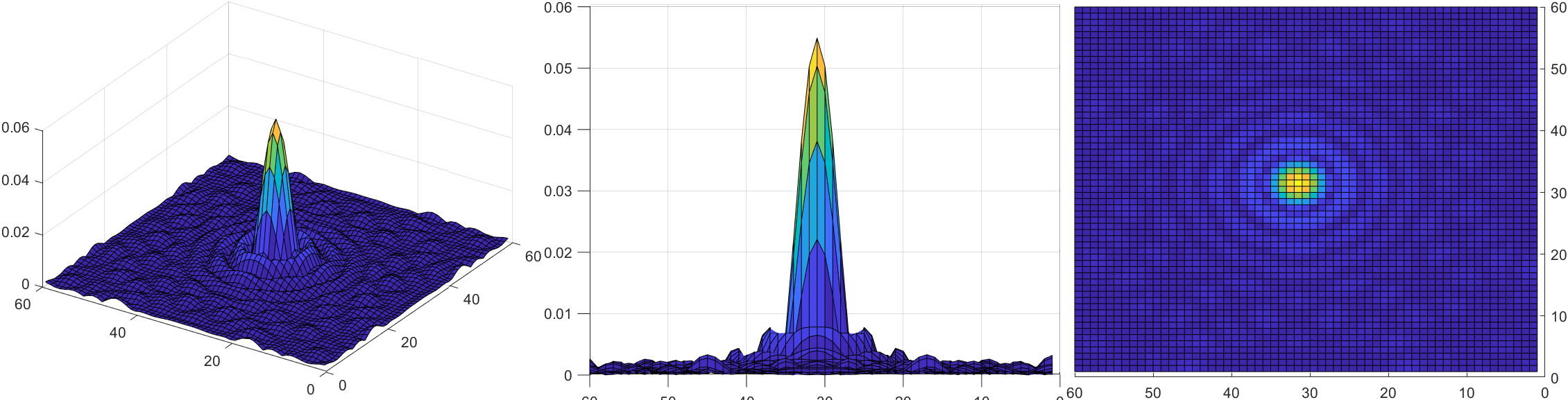##### 2.2. 巴特沃斯低通滤波器(Butterwoth Low Pass Filter)

巴特沃斯低通滤波器的冲激函数定义为： H ( μ , ν ) = 1 1 + [ D ( μ , ν ) / D 0 ] 2 n H(\mu,\nu)=\frac{1}{1+[D(\mu,\nu)/D_0]^{2n}} H ( μ , ν ) = 1 1 + ( 2 − 1 ) [ D ( μ , ν ) / D 0 ] 2 n H(\mu,\nu)=\frac{1}{1+(\sqrt{2}-1)[D(\mu,\nu)/D_0]^{2n}}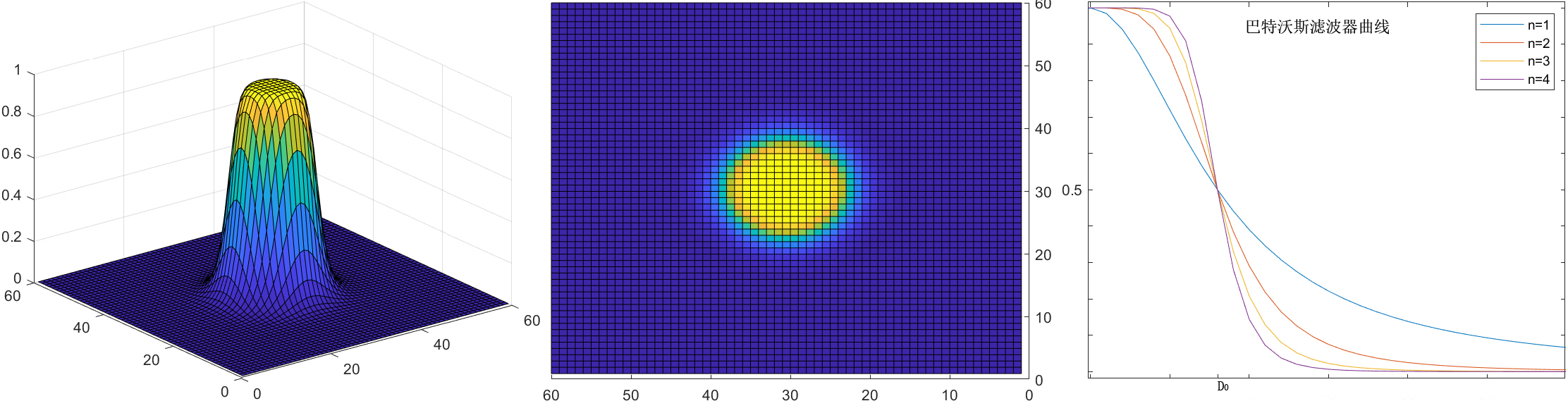根据图示可以看出，巴特沃斯低通滤波器与理想圆形低通滤波器最大的区别就在于，理想圆形低通滤波器有一个明显的阶跃界限，而巴特沃斯低通滤波器则是平滑变化的过渡带。而且经巴特沃斯低通滤波器处理的图像没有明显的振铃效应，这是过滤器在低频和高频之间平滑过渡的结果。

巴特沃斯低通滤波器的空域图像：
可以与上图理想圆形低通滤波器的空域图像对比，振铃效应减弱很多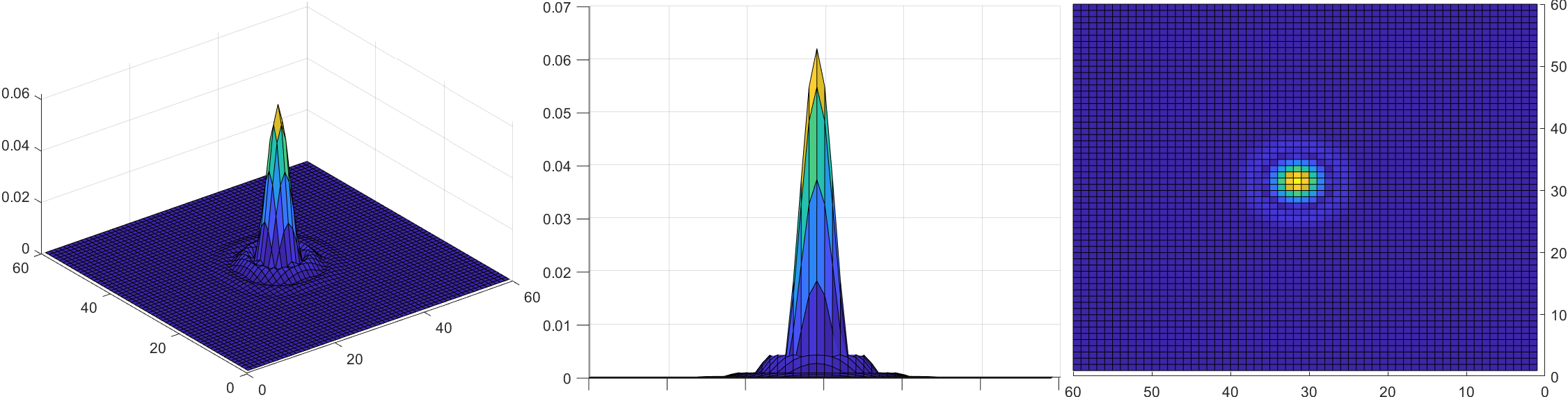##### 2.3. 高斯低通滤波器

高斯低通滤波器的冲激函数定义为： H ( μ , ν ) = e − D 2 ( μ , ν ) / 2 σ 2 H(\mu,\nu)=e^{-D^2(\mu,\nu)/2\sigma^2}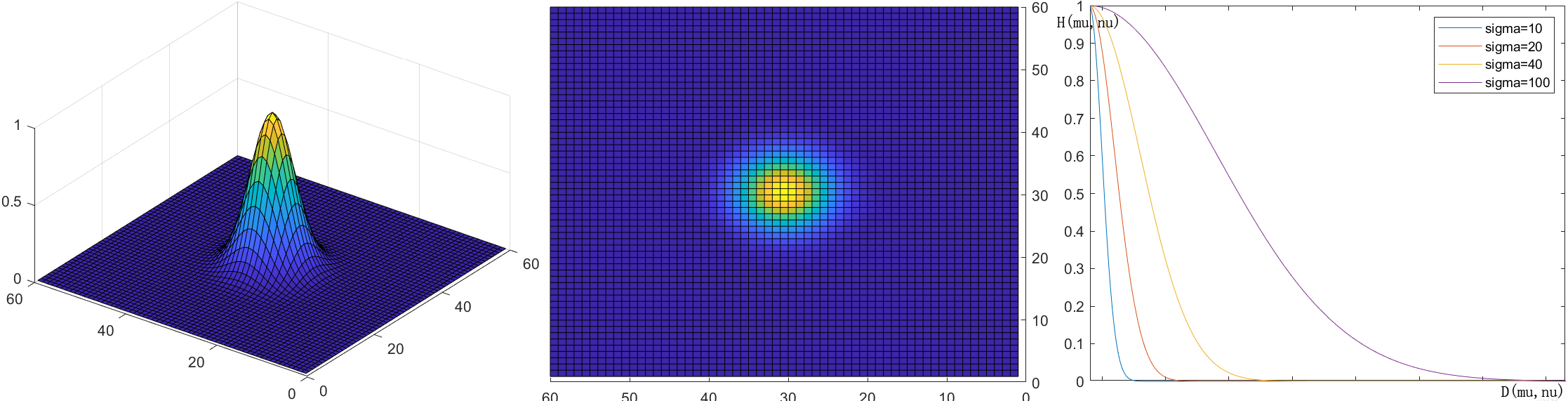高斯滤波器会把陡峭的图像边缘进行拓展，这样做虽然在一定程度上模糊图像，但却可以平滑图像、增强图像效果，使图像更易于辨认，例如：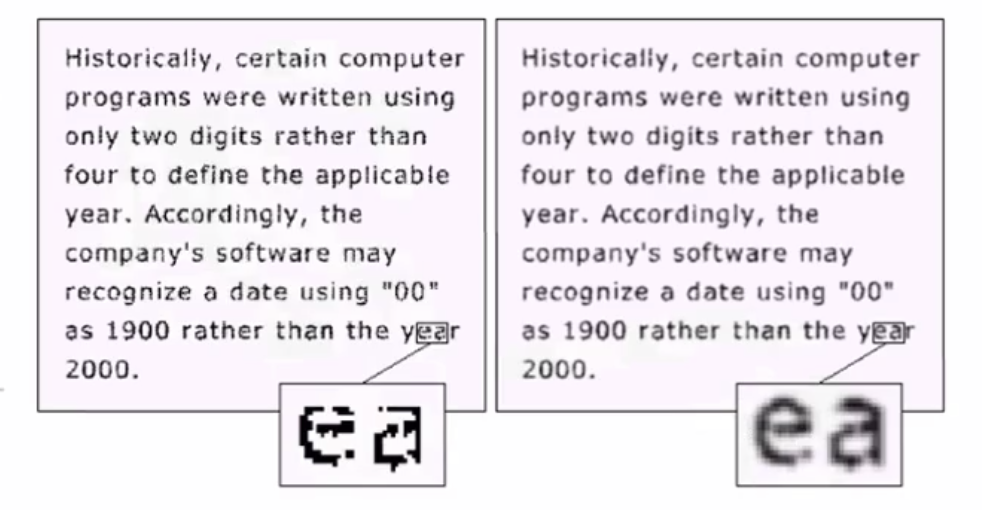### 3. 高通滤波器

高通滤波器与低通滤波器相对应，其数学表达式为： G ( μ , ν ) = F ( μ , ν ) H H P ( μ , ν ) G(\mu,\nu)=F(\mu,\nu)H_{HP}(\mu,\nu) 其中 H H P ( μ , ν ) = 1 − H L P ( μ , ν ) H_{HP}(\mu,\nu)=1-H_{LP}(\mu,\nu) ，1指的是整个频谱， H L P ( μ , ν ) H_{LP}(\mu,\nu) 指的就是低通滤波器

因此，低通滤波器中的常用滤波器经过简单变换之后就可以变为高通滤波器，常用的有：

1. 理想圆形高通滤波器 H H P ( μ , ν ) = { 0 , D ( μ , ν ) ⩽ D 0 1 , D ( μ , ν ) ⩾ D 0 ， D 0 为 截 止 频 率 H_{HP}(\mu,\nu)=\begin{cases} 0, & D(\mu,\nu)\leqslant D_0 \\[2ex] 1, & D(\mu,\nu)\geqslant D_0 \end{cases}，D_0为截止频率
2. 巴特沃斯高通滤波器 H H P ( μ , ν ) = 1 1 + [ D 0 / D ( μ , ν ) ] 2 n H_{HP}(\mu,\nu)=\frac{1}{1+[D_0/D(\mu,\nu)]^{2n}} H H P ( μ , ν ) = 1 1 + ( 1 − 2 ) [ D ( μ , ν ) / D 0 ] 2 n H_{HP}(\mu,\nu)=\frac{1}{1+(1-\sqrt{2})[D(\mu,\nu)/D_0]^{2n}}
3. 高斯高通滤波器 H ( μ , ν ) = 1 − e − D 2 ( μ , ν ) / 2 σ 2 H(\mu,\nu)=1-e^{-D^2(\mu,\nu)/2\sigma^2}
4. 指数高通滤波器 H H P ( μ , ν ) = e − [ D 0 / D ( μ , ν ) ] n H_{HP}(\mu,\nu)=e^{-[D_0/D(\mu,\nu)]^n}
5. 梯形高通滤波器 H H P ( μ , ν ) = { 0 , D ( μ , ν ) ⩽ D 1 D ( μ , ν ) − D 1 D 0 − D 1 , D 1 < D ( μ , ν ) < D 0 1 , D ( μ , ν ) ⩾ D 0 ， D 0 为 截 止 频 率 H_{HP}(\mu,\nu)=\begin{cases} 0, & D(\mu,\nu)\leqslant D_1 \\[2ex] \frac{D(\mu,\nu)-D_1}{D_0-D_1}, & D_1 < D(\mu,\nu)< D_0 \\[2ex] 1, &D(\mu,\nu)\geqslant D_0\\ \end{cases}，D_0为截止频率

### 4. 频域增强与空域增强

频域增强与空域增强在本质上是一致的，在空域增强中，当空域增强模板 h ( x , y ) h(x,y) 是相对于中心点对称时，其称为空域卷积模板，满足卷积表达式： g ( μ , ν ) = f ∗ h = ∑ x = 0 N − 1 ∑ y = 0 M − 1 f ( x , y ) h ( μ − x , ν − y ) g(\mu,\nu)=f*h=\sum_{x=0}^{N-1}\sum_{y=0}^{M-1}f(x,y)h(\mu-x,\nu-y) 变换到频域增强的形式只需对 g ( μ , ν ) g(\mu,\nu) 进行傅里叶变换即可

在实践中，空域增强比频域增强更常用，主要是因为其易于实现且执行速度快，频域增强一般用于空域增强难以达成的领域，例如图像压缩等等

展开全文图像增强 数字图像处理 滤波器
• Chapter 频域图像增强PPT教案.pptx
•matlab
• 对图像进行图像增强处理，包括空域和频域的一些方法
• Chapter 频域图像增强PPT学习教案.pptx
• ## 频域图像增强-锐化

千次阅读 2019-08-06 20:59:02
图像增强技术根据增强处理过程所在的空间不同，可分为基于频域的算法和基于空域的算法两大类。基于频域的算法是在图像的某种变换域内对图像的变换系数值进行某种修正，是一种间接增强的算法，把图像看成一种二维信号...
• 数字图像处理的一个作业，包含理想高通 巴特沃斯高通 高斯高通的频域增强的作业
• 图像增强频域滤波matlab代码数字图像处理技术 使用 Matlab 创建的数字图像处理技术 空间和频域滤波 本节涉及在空间和频域中用于图像处理的不同滤波方法的实现。 低光图像对比度增强 本节涉及用于低光图像图像处理的...
• 第4章 频域图像增强 图像的频域分析 频率域滤波 频率域平滑低通滤波器 频率域锐化高通滤波器 4.8 频率域平滑滤波 第4章 频率域滤波 图像的平滑除了在空间域中进行外也可以在频率域中进 行由于噪声主要集中在高频部分...
• 1． 给定一幅图象，画出其谱分布的平面图像，并对谱图像内容进行说明。 2． 频率域高通和低通滤波器设计，并以各种视图（立体图、平面视图、截面图）显示。 3． 给出一幅房屋图象，用高通滤波的方法求其边缘。
• 图像几何变换图像频域增强图像变换的目的傅里叶变换空间频率的理解如何看频谱图从频域变回空间域： 图像频域增强 图像变换是图像频域增强技术的基础，也是变换域分析理论的基础，图像频域增强基于图像信号的频域...傅里叶变换 数字图像处理 opencv
• 3.3 Python图像的频域图像增强-特殊高通滤波器 文章目录3.3 Python图像的频域图像增强-特殊高通滤波器1 算法原理1.1高频增强滤波器1.2高频提升滤波器2 代码3 效果 1 算法原理 特殊高通滤波器（高频增强滤波器、高频...opencv 计算机视觉 python
• 04图像频域变换及增强DFT，离散傅里叶变换，图像频率滤波
• 基于MATLAB的数字图像课程设计-图像频域增强高通滤波器算法设计.pdf
• 图像的处理可以直接在图像空间进行，也可以考虑将图像变换到其他空间再进行处理。...如果让不同频域范围内的分量受到不同的抑制，即进行不同的滤波，就可以改变输出图的频率分布，达到不同的增强目的。
• 频域中，图像增强则主要通过对空间频率（spatial frequency）进行操作来实现 与空间域滤波不同的是，频率滤波并不直接对图像像素数据进行操作，而是先通过快速傅里叶（FFT）变换将图像转换至频率域，经滤波处理后...
• 3.1 Python图像的频域图像增强-图像的傅里叶变换和反变换 文章目录3.1 Python图像的频域图像增强-图像的傅里叶变换和反变换1 算法原理2 代码3 效果 1 算法原理 图像的傅里叶变换和反变换（需要考虑图像旋转、平移时...opencv python 计算机视觉
• 本文档是基于图像增强技术的频域滤波算法研究,主要是关于图像增强频域滤波算法。图像增强
• 通过MATLAB进行频率域图像增强实验，注意文件和图片在同一目录下...